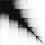# A different way to find the highest power

For a prime $$p$$ and $$n\in\mathbb{N}$$, you've probably seen this formula somewhere for the highest power of $$p$$ dividing $$n!$$:

$\displaystyle \sum _{ i\ge 1 }^{ \quad }{ \left\lfloor \frac { n }{ { p }^{ i } } \right\rfloor }$

In fact, there's a much more surprising formula (let's face it, this one isn't that hard to derive): suppose $n={ ({ b }_{ 0 }{ b }_{ 1 }{ b }_{ 2 }...{ b }_{ r }) }_{ p }$, or

$n={ b }_{ 0 }{ p }^{ r }+{ b }_{ 1 }{ p }^{ r-1 }...+{ b }_{ r }$

and let $\delta (n)$ be the digit sum of $n$ base $p$. Then the highest power of $p$ dividing $n!$ is

$\displaystyle \frac { n-\delta (n) }{ p-1 }$

Wow. That's a heck of a lot shorter, isn't it? But why does this even work? Let's prove it below...

$\frac { n-\delta (n) }{ p-1 } = \frac { n-({ b }_{ 0 }+{ b }_{ 1 }+...{ b }_{ r }) }{ p-1 } =\frac { \displaystyle \sum _{ k=0 }^{ r }{ { b }_{ k }({ p }^{ r-k }-1) } }{ p-1 }$

Then factor ${p}^{r-k}-1$ (by difference of nth powers) and cancel the $p-1$ in the denominator. This results in

$\displaystyle \sum _{ k=0 }^{ r }{ { b }_{ k }\left( 1+p+{ p }^{ 2 }...{ p }^{ r-k-1 } \right) } = b_{r-1}+(b_{r-2}p+b_{r-2}) \dots$

Now write this sum out - term by term - in columns (not a typo, I mean columns - there's a trick here):

$\displaystyle \sum _{ k=0 }^{ r }{ { b }_{ k }\left( \sum _{ j=0 }^{ r-k-1 }{ { p }^{ j } } \right) } =({ b }_{ r-1 }+{ b }_{ r-2 }p+\dots+ { b }_{ 0 }{ p }^{ r-1 })$ $\quad\quad\quad\quad\quad\quad\quad\quad\quad\quad\quad+(b_{r-2}+ \dots + b_0 p^{r-2})$ $\quad\quad\quad\quad\quad\quad\quad\quad\quad\quad\quad\quad\vdots$ $\quad\quad\quad\quad\quad\quad\quad\quad\quad\quad\quad\quad\quad\quad\quad\quad\quad\quad +b_0$

Okay, while each term was written by column before, let's look at the rows now. In fact, each row is equal to a term in this familiar expression:

$\displaystyle \sum _{ k=1}^{ r }{ \left\lfloor \frac { n }{ { p }^{ k } } \right\rfloor }$

because each time you divide by $p$ again one term in the base $p$ expansion vanishes (that associated with $p^0$) and the others go down an exponent of $p$. So, we have

$\displaystyle \sum _{ k=1}^{ r }{ \left\lfloor \frac { n }{ { p }^{ k } } \right\rfloor } = \displaystyle \frac { n-\delta (n) }{ p-1 }$Note by Dylan Pentland
5 years, 10 months ago

This discussion board is a place to discuss our Daily Challenges and the math and science related to those challenges. Explanations are more than just a solution — they should explain the steps and thinking strategies that you used to obtain the solution. Comments should further the discussion of math and science.

When posting on Brilliant:

• Use the emojis to react to an explanation, whether you're congratulating a job well done , or just really confused .
• Ask specific questions about the challenge or the steps in somebody's explanation. Well-posed questions can add a lot to the discussion, but posting "I don't understand!" doesn't help anyone.
• Try to contribute something new to the discussion, whether it is an extension, generalization or other idea related to the challenge.
• Stay on topic — we're all here to learn more about math and science, not to hear about your favorite get-rich-quick scheme or current world events.

MarkdownAppears as
*italics* or _italics_ italics
**bold** or __bold__ bold
- bulleted- list
• bulleted
• list
1. numbered2. list
1. numbered
2. list
Note: you must add a full line of space before and after lists for them to show up correctly
paragraph 1paragraph 2

paragraph 1

paragraph 2

[example link](https://brilliant.org)example link
> This is a quote
This is a quote
    # I indented these lines
# 4 spaces, and now they show
# up as a code block.

print "hello world"
# I indented these lines
# 4 spaces, and now they show
# up as a code block.

print "hello world"
MathAppears as
Remember to wrap math in $$ ... $$ or $ ... $ to ensure proper formatting.
2 \times 3 $2 \times 3$
2^{34} $2^{34}$
a_{i-1} $a_{i-1}$
\frac{2}{3} $\frac{2}{3}$
\sqrt{2} $\sqrt{2}$
\sum_{i=1}^3 $\sum_{i=1}^3$
\sin \theta $\sin \theta$
\boxed{123} $\boxed{123}$

## Comments

Sort by:

Top Newest

Wow! Nice, let me see whether I can apply to this question.

- 5 years, 10 months ago

Log in to reply

×

Problem Loading...

Note Loading...

Set Loading...